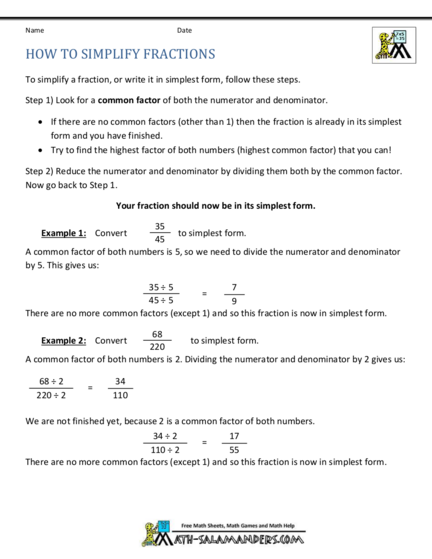# Reducing Fractions to Lowest Equivalent FormContributed by:This pdf covers steps to simplify a fraction to the lowest form by finding common factors and removing them. Equivalent fractions are the fractions that have different numerators and denominators but are equal to the same value.
1. Name Date
HOW TO SIMPLIFY FRACTIONS
To simplify a fraction, or write it in simplest form, follow these steps.
Step 1) Look for a common factor of both the numerator and denominator.
• If there are no common factors (other than 1) then the fraction is already in its simplest
form and you have finished.
• Try to find the highest factor of both numbers (highest common factor) that you can!
Step 2) Reduce the numerator and denominator by dividing them both by the common factor.
Now go back to Step 1.
Your fraction should now be in its simplest form.
35
Example 1: Convert to simplest form.
45
A common factor of both numbers is 5, so we need to divide the numerator and denominator
by 5. This gives us:
35 ÷ 5 7
=
45 ÷ 5 9
There are no more common factors (except 1) and so this fraction is now in simplest form.
68
Example 2: Convert to simplest form.
220
A common factor of both numbers is 2. Dividing the numerator and denominator by 2 gives us:
68 ÷ 2 34
=
220 ÷ 2 110
We are not finished yet, because 2 is a common factor of both numbers.
34 ÷ 2 17
=
110 ÷ 2 55
There are no more common factors (except 1) and so this fraction is now in simplest form.